# Find the regression lines for the men’s and women’s data.### Precalculus: Mathematics for Calcu...

6th Edition
Stewart + 5 others
Publisher: Cengage Learning
ISBN: 9780840068071### Precalculus: Mathematics for Calcu...

6th Edition
Stewart + 5 others
Publisher: Cengage Learning
ISBN: 9780840068071

#### Solutions

Chapter 1, Problem 11P

a.

To determine

## Find the regression lines for the men’s and women’s data.

Expert Solution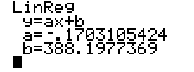### Explanation of Solution

Calculation:

Select the men’s data and put it as input in calculator then press STAT button then select EDITING.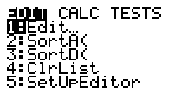Now input year data in L1 column and men’s 100m time in L2 column.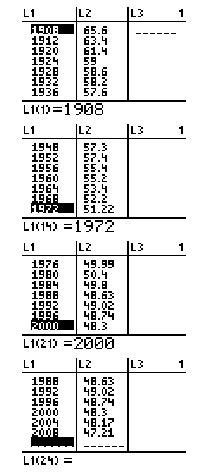For regression line press STAT go to CALC menu, and select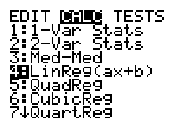The display shows slope intecept form of line,Apply similar method for women’s data and get regerssion line as,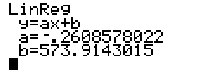Hence, the regression line are,b.

To determine

### Sketch both regression lines on the same graph.

Expert Solution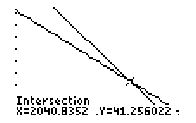### Explanation of Solution

Calculation:

Let graph the lines in viewing rectangle [1900,2012]by[340,400] with the help of graphing calculator, then set the viewing window to 1980x2012,340y400 then we set Xsc1=2,Ysc1=10 .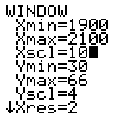Now evaluate intercepts with CALC “intercept”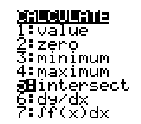Now graph of lines will be,Hence, both regression lines on the same graph is plotted.

### Have a homework question?

Subscribe to bartleby learn! Ask subject matter experts 30 homework questions each month. Plus, you’ll have access to millions of step-by-step textbook answers!